HistoryMathematiciansAll formulasNum. approx.SoftwaresMisc. math.DigitsPoetryPapers/videosDelirium !Pi-DayImagesMusicLinksBibliography Boris Gourévitch The world of Pi - V2.57 modif. 13/04/2013HomeVersion historyGuestbookWho I amSome pictures (fr)AcknowledgementsLast modificationsContact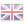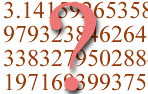Pi's decimals and Statistics

This last section of the triology of randomness is on the tireless research to, AT LAST, find something particular to Pi ! Because since we have been interested in our favourite constant, and invade its decimal that up till then have been protected, we need to admit that this sequence of digits, as if plucked from nowhere, is the most intriguing!
Ok, Pi is irrational, so we won't find the same periodic sequences...
But with a few not so complicated tools, we could maybe find other patterns??
Like the previous three, this page is meant to enrich itself with my collection, and of your eventual contributions!
Don't hesitate if you have a few more ideas or examples, tell me...

Here are the paragraphes tackled in order :

A - The fractal dimension

1 - Estimation of the fractal dimension of a curve
2 - The rescale range method

B - Testing the decimals

1 - Khi-squared
2 - The poker test
3 - Sums of decimals

C - Other approach

1 - Khintchine's Constante
2 - And so ??????

D - Bibliography

A - The Fractal Dimension

So, after a page on random theory, it is known that we know nothing at all on the decimals of Pi (in theory)!
Ok, but if we look directly at the decimals now, can we not find some strange structure somewhere, which are a bit out of the ordinary ?
Because, there must be something hidden behind the billions of decimal we have access to! As Gregory Chudnovsky said, it would be a disaster if the decimals would show nothing before the 1077 decimals that we are in thoery cabable to calculate if we used every atom in the universe ! And we are quite far, you can imagine, since we only found the 1011 decimals in september 2000 (206 billion).

The Chudnovsky wrote in 1991 that the decimals of Pi appeared to be more random than generated by a human, but still maybee not enough random!
The logarithm law iterate by Chung described on the page dedicated to randon
phenomenon suggested to the same Chudnovsky to consider a random walk with the sequence of decimals (remember that with Donsker's theorem, the sum of a random walk converge roughly towards a Brownian motion). From there, we can construct fractals objects from the decimals of Pi, and why not measure their fractal dimendsions! Of course, that a great idea!
The fractal dimension of a classical procedure like the Brownian motion is 1.5.
Vanouplines, from Vrije Universiteit te Brussel (Belgium), demonstrated that the dimension of Pi is also very near 1.5.
The fractals are a very rich domain of mathematics, and my page on Mandelbrot shows that we can even find Pi in the Mandelbrot set!
The definition of a fractal, given by Mandelbrot himself in his booke "The fractal geometry of nature" (1983) is, as Weyl would say "a foggy fog"! :

A fractal object is by definition an object whose Hausdorff-Besicovitch dimension is stricly greater than its topological dimension"

hum, thanks...
Mandelbrot states a bit later on that he still thinks that it would be better with no definition... :-)

On the subject of dimension, things are a bit more intuitive in euclidian spaces: a point has of course dimenion 0, a line has dimension 1, a plane has dimension 2, a volume has dimension 3, etc...
Only, all those dimensions are integers... Can you imagine some curves with rational dimension, or even real?
In fact, in a intuitive way the plane is full of an infinite number of lines. The line has no width, but if we make it curve in all direction, more and more tightly, the line will start occupying loads of space, and so it's fractal dimension is greater than 1, the limit hence being the plane of dimension 2. In short, the more the curve is crooked, and this no matter how much you zoom, more will the dimension of the curve be greater than 1.

A.1 - Estimation de la dimension fractale d'une courbe

Here we are using the "box count" method which makes use of the second definition of fractal objects by Mandelbrot, as vague as the previous one, but more
intuitive :

A fractal object is a rough or fragmented geometric shape that can be split into parts, each of which is (at least approximately) a reduced-size copy of the whole.

Anyway, at whatever scale you choose, the object appears to be the same...
Take a coast on a sea map... this is a good example of a fractal curve!
So, if we take some squared paper of 1 millimetre, then a squared paper of 2 millimeters, and 4mm and finnaly 8mm. You understood it, the different sizes of the squares will be used to compare the drawing at different scales.... This is consistant with the form the different zoom used will produce which determines the complexity degree of a fractal object.

We take for each of those squared paper the same rectangular zone and we put on it the coast drawing. We then count the number of squares crossed by the curve and we take down the result in a table like the one that follows: (fictive data taken from )

 Size of squares Number of squares crossed Size of squares*Number of squares crossed (coast length) Decimal Logarithm of the squares' size Logarithm of the coast length Fractal dimension 1 6998 6998 0 3.845 1.39 2 2679 5358 0.301 3.729 1.35 4 1054 4216 0.602 3.625 1.32 8 424 3392 0.903 3.530 1.31 16 171 2736 1.204 3.437 1.33 32 68 2176 1.505 3.338 1.33 64 27 1728 1.806 3.238 1.44 128 10 1280 2.107 3.107

The coast length is longer when the precision is greater as we then find more twist on the coast. We can then see that the object can not be of high fractal dimension, but still it is neither 1.
By graphing the log of the coast length (5th column) against the log of the square size, we get a straight decreasing line whose gradient is 1 - D, where D is the fractal dimension. Intuitivly, this is expected. In fact if the gradient was 1, the coast length would be proportional to the precision wanted, hence there would have been no fractal criteria in the curve and D=0 in this case. Here, the gradient is negative as shown in the following figure, which means that the square size increase faster than the visible coast length decreases. In the above table, the dimension is evaluate for reach gradient between two points
With a gradient of -0.339 for the straight line, we get an average fractal dimension of D=1-(-0.339)=1.339.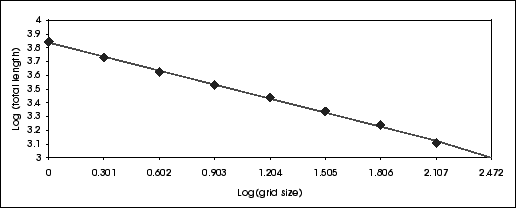So, now that we know what is a fractal dimension, the idea is to draw the random walk of the decimals of Pi and estimate it's fractal dimension.
Here is the graph where each decimals is linked to the previous and the next by a line :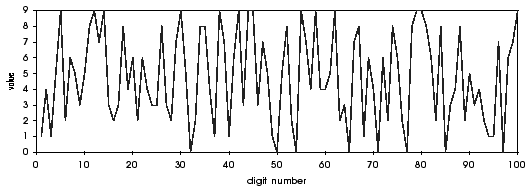Ok, but there still a major problem... It's that in the coast, the scale was the same in abscissa and ordered. Here, it's quite the opposite.... ouch!

A.2 - Rescaled range method

But our friends the mathematicians thought about this problem and came up with the rescale range method in the 660s with Hurst, Mandelbrot and Wallis.
Hurst gave the following notations: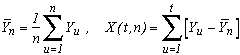where Yi is the ie decimale of Pi-3.
He then define the two statistics :And the best, is that our friend Hurst noticed that the statistic R/S often took a surprising form! In an emperical way, we get :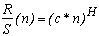where c is a constant often taken to be equal to 1/2 and H is Hurst's exposant. This allows to keep the abscissa scale and to reduce the cummulated sum of the digits observed according to this scale.
But how to link it back to the fractal dimension ?
Well in fact, the relation between the fractal dimension D and Hurst's coefficient H is

D=2-H

This is intuitevely understood since the higher H is, the more does the ration R/S ingrease rapidly with n. This means that the maximum amplitude increase more and more quickly compared with the variation, or more mundanely that the variation increase more slowly than the maximum amplitude. But this is nothing else but (for me!) the fact that we "zoomed" on the curve but that the variation did not follow, and so the fractal dimension decreases.

Still with our hands, an exposant H between 0.5 and 1 shows persistence sign in the curve, that is if it has experienced an increase over a certain period, this increase will probably carry on the next period. For an exposant less than 0.5 it's exactly the opposite with some agitations more chaotic and less predictable, this is logical since then the fractal dimension  increase. It is therefore not surprising that for the procedure of finite independant variance, this exposant H if 1/2, (for example the Brownians mothion, which shows that their dimensial fractal is 1.5).
Anyway, and it's amazing to find Pi in this area, Feder showed in 1988 that for this procedur, we have exactly:Incredible, no ??
For most of the natural phenomenon, H=0.72 hence D=1.28.
And for our good friend Pi ?
Instead of using the decimals of Pi, the average of the cumulated decimals is a more "continous" curve, which looks more like a random procedure. By denoting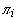to be the ie decimale of Pi, we therefore consider the procedur :being of course with digits between 0 and 9, the average expected is hence 4.5 and so 2-9 should be close to zero on average.
In fact, on the first 100 decimals, we get the following graph of Sp :Well if this is not a beautiful procedur ?!
For 1.25 million decimals, we get the following curve with fractal dimension:A clear tendance, which is provided by the curve the fractal dimension is 1.45. Yes, it's not a procedure with random increasement completely independant like the Brownian motion! There exists a little persistance  (H=0.55), but to find out which one... hmmm...
So, the whole fractal dimension is a good indicator to tell us that obviously, something is going wrong! But it doesn't tell us what.... Let us examine a bit more the empirical spread of the decimals :

B - Testing the decimals

The fact that the decimals of Pi have no problem passing the most classic tests like Khi-squared, the poker test, and the arctan law does not help....
Euh.... by the way, what's all this ?
Ok, to tell the truth, the mathematicians have not found much - I don't blame them ! :-) - on the spread of decimals by studying the number Pi itself, through its appearance in the formulae or theories... The method, is then no more probabilistic but statistic, involves inversing the previous method and this time starting from the decimals to find singularity to Pi. And we have to admit that it's not that simple....

1 - Khi-squared

It's the most classical, and one of the weakest.... the statisticians tend to say that everything goes through a Khi-squared :-)
It's a statistic that works out the sum of the squared difference of the frequences observed with the frequence expected. Under the hypothesis that the data does follow the expected result, it follows as it names stated a Khi-squared with freedom degree of n-1 where n is the number of frequence we are considering :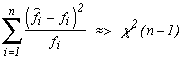fi is the expected frequence andWe don't have a freedom degree of n  since the last frequence has to be known due to the others (the sum of the frequence is the number of decimals used, hence known!). Only n-1 frequences actually influence the statisctic calculation which is why we attribute a freedom of degree n-1 to the law. All of this can be proven of course, but it's not the idea of this page or even this site !
Then all is needed is to compare the value obtained with the value taken from the associated law. If this value is less than a fractal order of 0.95, this means that probability observed in nature of the value Khi-squared greater than this statistic is greater than 5%, etc... In short, this would imply that our constant has nothing exceptional....
And guess what happens!
Of course, nothing.... :-)
Here are the frequence observed for the first 200 billion decimales of Pi-3 :

 Digit Appearance in Pi Appearance in 1/Pi 0 20000030841 19999945794 1 19999914711 20000122770 2 20000136978 20000060451 3 20000069393 20000182235 4 19999921691 19999876817 5 19999917053 19999977273 6 19999881515 19999911742 7 19999967594 20000001035 8 20000291044 19999927489 9 19999869180 19999994394 Statisctics of Khi-squares 8.09 4.18

Those two statistics correspond to fractal order of 0.53 and 0.9 respectively. That is that we get respectively 53% and 90% observation in the statistic nature to take a greater value... Basically, nothing exceptional!
Note that those two statistics are calculated only on the first 200 billion decimals, so it's only to see if anything goes wrong! Because there could be a few variation and there are no reason for a decimal to appear more often than others, or less. So it's not very powerful !
Kanada, who calculate those 200 billions decimals, did the test by blocks of decimals, the files are available at ftp://pi.super-computing.org/. The Khi-squared test for the successive splitting of 10 blocks the 6 billions decimals available localy. But really nothing special...

So, let us continue our invistigation !

B.2 - The poker test

This statistic is a bit better than the Khi-square since it is interested not in each decimals, but in combination between decimals.
We put the decimals in blocks of 5, and in each of those blocks, we look to see what poker combination we find.
For this block, we either ger different decimals, or a pair, or three of a kind, a double pair, four of a kind, five of a kind (euh, quite hard in poker), or a full house...
This kind of test can be more funny than serious ! However, in nature we expect to find a certain number of paires, three of a kind, etc... This test is of higher level than the Khi-squared test in the regularity of the decimals combination frequence.
For 200 000 poker's hand for example, the expected frequences are the following :

 Combinaisons Fréquences attendues Décimales différentes ABCDE 60480 Paires AABCD 100800 2 Paires AABBC 21600 Brelans AAABC 14400 Full House AAABB 1800 Carré AAAAB 900 Quinte AAAAA 20 Total 200000

To obtain those expected frequence, we just need to count the possible cases over the favourable case as we say in probability. Let us take an example with the 4 of a kind:
we have 1 in 10 chance of getting exactly A (10 decimales), an other 1 in 10 to get another A and so on, so by argument, we have a 1/104 chances to get AAAA. But it could be an A like it could be a C or an other digit, so we have 10 different possible cases, we multiply the total by 10, this gives 1/103. Then, we need to get an other digit that is not A, there are only 9 remaining, so we have a 9 in 10 chance to get it and so we multiply the total by 9/10. Finally we need to place this B with the A, since there are 5 places, we have a choice of 5 different possibility so we multiply the total by 5.
In the end we get:

5*9/(10*103)=0.0045

Since we have 200 000 draws, the number of 4 of a kind expected is 200 000*0.0045=900, which is what was expected. Working methodically (so not like me), we should always get it, but it's true that it's not that easy and that a bit of practise is needed !
The principle is then to do a Khi-squared test on the result to compare them with the expected result. Kanada's team did those various test on the record of 6 billion decimals. We general group together the 4 of a kind and 5 of a kind due to the weak frequence of 5 of a kind, but this was not done in the following test. The Khi-squared considered hence has a freedom degree of 6, and we of course did it on 1 200 000 blocks of 5 decimals :

` DECIMALE         1          2          3          4          5          6          7          8          9         10|  TOTAL       EXPECTED ---------------------------------------------------------------------------------------------------------------------------------------------- Déc diff  36294173   36290069   36290127   36286820   36290298   36289575   36294505   36289984   36281969   36282987|  362890507   362879996.98 Paires    60475840   60476864   60485069   60484354   60477375   60474120   60476577   60473797   60485057   60486104|  604795157   604799994.96 2 Paires  12956498   12958229   12954176   12962149   12961317   12963422   12962341   12963379   12959383   12960093|  129600987   129599998.92 3 same     8643856    8641687    8639415    8636938    8640244    8639352    8635473    8640375    8642190    8641230|   86400760    86399999.28 Full House 1078694    1080546    1079458    1079213    1079216    1080368    1078612    1080174    1078744    1078985|   10794010    10799999.91 4 same      539027     540460     539627     538583     539598     541309     540416     540236     540532     538638|    5398426     5399999.95 4 same       11912      12145      12128      11943      11952      11854      12076      12055      12125      11963|     120153      120000.00 ----------------------------------------------------------------------------------------------------------------------------------------------  KHI2         7.98       3.27       5.10       6.36       1.46       6.67       6.74       2.03       5.30       5.99|      4.33  (FRACTAL     0.25       0.77       0.54       0.4        0.96       0.36       0.35       0.92       0.51       0.44|      0.64  APPROCHE)`

So there was a decimal test, then a total test (that a good use of Khi-squared, we can test anything!). And we can see that there are nothing to be noticed, no fractal comming anywhere need 0.05...
At the most the gaps between the digit 5 is considered too weak ! :-)

Aie aie aie, this doens't simplify things, still nothing...
But let us push forward !

B.3 - Sum of 5 decimals

As the name states, this test cuts the decimals in blocks of 5 and calculate the sum of each block, This test is meant to show parts where for example there are more frequently high decimal would make higher sum more common than in nature, etc...
The expected results are follow up on the multinomial laws. But it seems simplier to find the result using good old logical reasoning...
You know that we estimate a probability by wanted cases on possible cases. This is a way to proceed
Let us start easily: take a block of 5 digits, so that their sum be 0, all 5 digits must be 0, so there are only one possibility! This is the number of wanted case. On the other hand, each of the 5 digits have 10 different possible value, so the number of possible case is 10*10*10*10*10=105. So the probability that the sum is 0 is 1/105. Since we have 1.2 billion blocks, this give

1.2*109/105=12000

expected case, simple.
Ok, a second example not so trivial now because i know you've warmed up !
In the third case, we need the sum of the 5 digits to be 2. We get several cases :
Either two digits are 1 and we get C(5,2)=10 (2 choose 5, C(n,k)=n!/(k!(n-k)!) ) ways to choose them between the 5 digits. Using one of the multinomial laws M(5,0.1,0.1,...) we get 5!/(3!2!0!0!...)*1/(103102100100...)=10/105 the probability for this event, for advanced reader who want to speed up.
Or a digit is 2 and the four others are 0, this can be in 5 different ways depending where you put the 2. With the multinomial, 5!/(4!1!0!0!...)*1/(104101100100...)=5/105 is the probability of this event. In the end we have 15 different wanted case hence a probability for this event of 15/105 that we can get through two method, either by hand or by multinomial.
The expected number of "SUM=2" on 1.2 billion block is therefore

1.2*109*15/105=180000

I tell you it gets funny very quickly to see if we have a little logic in counting or not, try it!
Still on the 6 billions decimals and breaking them in blocks of 600 millions decimals, Kanada's team got the following results :

`  BLOC =          1          2          3          4          5          6          7          8          9         10|   TOTAL      ATTENDU ------------------------------------------------------------------------------------------------------------------------------------------------ SUM=  0       1216       1197       1193       1250       1200       1176       1196       1214       1152       1233|      12027      12000.000 SUM=  1       5923       6023       5957       5967       6017       6028       6077       6045       6047       5975|      60059      60000.000 SUM=  2      17848      18002      17896      17914      18100      17835      17874      18127      17887      17937|     179420     179999.998 SUM=  3      42128      41943      41885      42180      41659      41987      41697      42120      41935      42088|     419622     419999.997 SUM=  4      84093      83998      83899      83694      83827      84749      83906      84146      83647      82995|     838954     839999.993 SUM=  5     150933     151285     152040     150889     150914     151440     151465     151357     151106     150406|    1511835    1511999.987 SUM=  6     252052     251554     252425     252377     251639     251762     251674     252596     252215     252220|    2520514    2519999.979 SUM=  7     395189     396146     395922     396137     396044     395779     396032     396210     396185     395973|    3959617    3959999.967 SUM=  8     593637     592684     593729     594333     593971     593748     594449     594366     595328     593856|    5940101    5939999.951 SUM=  9     858470     858342     858321     856921     858350     859226     857611     857056     857478     858960|    8580735    8579999.929 SUM= 10    1194437    1195429    1194514    1194536    1193553    1194870    1194976    1194134    1193540    1194598|   11944587   11951999.900 SUM= 11    1605266    1607515    1608231    1607348    1608394    1605279    1609486    1608762    1609340    1609442|   16079063   16079999.866 SUM= 12    2091618    2095708    2094196    2092715    2093180    2091291    2095945    2093030    2093830    2092915|   20934428   20939999.826 SUM= 13    2648030    2646321    2645322    2643188    2647514    2646881    2644930    2645962    2643577    2643418|   26455143   26459999.780 SUM= 14    3252283    3252822    3249652    3253274    3250272    3253381    3249525    3252776    3253078    3250408|   32517471   32519999.729 SUM= 15    3899546    3898175    3893160    3893092    3898171    3894389    3895990    3897963    3895193    3892981|   38958660   38951999.675 SUM= 16    4551996    4551347    4554651    4553603    4553925    4552191    4556515    4553971    4552146    4553568|   45533913   45539999.621 SUM= 17    5201733    5201032    5201711    5203465    5201273    5206295    5205467    5202503    5205968    5204304|   52033751   52019999.566 SUM= 18    5809804    5806104    5812753    5808793    5810430    5805164    5806757    5809698    5808856    5811122|   58089481   58079999.516 SUM= 19    6335116    6335295    6334714    6340044    6337456    6338388    6334646    6335387    6333802    6337037|   63361885   63359999.472 SUM= 20    6753880    6755164    6757793    6756423    6757089    6754552    6754976    6757225    6758836    6754169|   67560107   67571999.437 SUM= 21    7048473    7047684    7054697    7049417    7047712    7050818    7055967    7052409    7047565    7049867|   70504609   70499999.413 SUM= 22    7195837    7204989    7194831    7199905    7198519    7203840    7196208    7201386    7198388    7201909|   71995812   71999999.400 SUM= 23    7203470    7201800    7195852    7197458    7200117    7198749    7199849    7195443    7198608    7197627|   71988973   71999999.400 SUM= 24    7050060    7047213    7049745    7048952    7051751    7051705    7051021    7045667    7046796    7052240|   70495150   70499999.413 SUM= 25    6761856    6760420    6761986    6763441    6754330    6755526    6758730    6761985    6763792    6756381|   67598447   67571999.437 SUM= 26    6335540    6335738    6337255    6332486    6337542    6333532    6337991    6336563    6336177    6338455|   63361279   63359999.472 SUM= 27    5808627    5808453    5807373    5807508    5808064    5810369    5804582    5805840    5805965    5810139|   58076920   58079999.516 SUM= 28    5202613    5202126    5204017    5200678    5197496    5202708    5204795    5201079    5205991    5202461|   52023964   52019999.566 SUM= 29    4555210    4553387    4553230    4555919    4557110    4552793    4553511    4554100    4554409    4553357|   45543026   45539999.621 SUM= 30    3895961    3892915    3894162    3894606    3894262    3894426    3892307    3894606    3894956    3895352|   38943553   38951999.675 SUM= 31    3256018    3250243    3251867    3249837    3253079    3249265    3253032    3249406    3252452    3253948|   32519147   32519999.729 SUM= 32    2644512    2648978    2647511    2648128    2643971    2644766    2643985    2645457    2645607    2644185|   26457100   26459999.780 SUM= 33    2093395    2095113    2096225    2095226    2092831    2094830    2093681    2095801    2093199    2095237|   20945538   20939999.826 SUM= 34    1607321    1609097    1608165    1609658    1608917    1608961    1606667    1607598    1608289    1608217|   16082890   16079999.866 SUM= 35    1195598    1193679    1193808    1195018    1196316    1196777    1195415    1195170    1195168    1193806|   11950755   11951999.900 SUM= 36     857619     858681     856824     858062     858473     859108     856967     857623     856698     857784|    8577839    8579999.929 SUM= 37     594438     594375     594600     593669     594880     595027     594359     594477     594675     593578|    5944078    5939999.951 SUM= 38     394990     395440     395415     397078     396760     396359     395443     396010     395884     395526|    3958905    3959999.967 SUM= 39     251920     251551     251452     252773     252682     252077     251600     252275     252268     251785|    2520383    2519999.979 SUM= 40     150416     151017     150658     150839     150496     150787     151427     151874     150468     151099|    1509081    1511999.987 SUM= 41      83539      84096      83381      84021      83994      84005      84573      83593      84225      84090|     839517     839999.993 SUM= 42      42301      41678      41976      42218      42204      41926      41479      41954      42097      42034|     419867     419999.997 SUM= 43      17972      18008      17838      17777      18371      17881      18101      17928      17898      18073|     179847     179999.998 SUM= 44       5958       6047       5932       5986       5964       6105       5937       5930       6026       6029|      59914      60000.000 SUM= 45       1158       1186       1236       1197       1181       1249       1179       1178       1253       1216|      12033      12000.000`

Hum, still nothing interesting.... hopeless...
Kanada's team did one more test, called the Gap test but I never understood it! If someone can explain to me? The files which explain it is on this adresse.

The arctan law test also remains a mystery for me and I haven't found any reference to it on the web which discusse it. Well since we can't invent everything yourself (!), I take my leave, I will wait for a nice soul to come an help me....

Let me say that numerous are the graphical method used to try and find regularity in the decimals. For example change the decimals into binary and put them end on end in a square to see if they make a picture. If the  landscape produced seems more regular thant if made by pure chance, the Chudnovsky have not found any satisfying explenation to this kind of phenomena...

Here is a quick tour of classical statistical method to analyse decimals

C - Other approaches

Quite a few crazy ideas have been passed in the domain of singularity research. A few ideas sometime are worth attention, like the one that consiste of using the knowledge of a number group in general to test if this group belong to a particular constant. A good example uses Khintchine's constant.

1 - Khintchine's Constant

Aleksandr Khintchine publishes in 1935 a little bookled on continued fractions, ("Continued Fractions", why complicate the title ?) in which he notes that the geometric mean of the coefficient of of a continue fraction tends to a certain constant, and this is often certain (except for a set of number with measurment null, the measure being Lebesgue one)....
In English and in clear (because this site is not a theory lecture on measure!), this means that this result is true except for a set of isolated numbers, with no continuity between them, even of infinite size (like N or Q). There exists of course a few exotic sets that did not fit this intuitive explination but that's not the point.
In general, he showed the following theorem :

Khintchine's theorem

Suppose that f(r) is a funtion of positive integer r and suppose that there exists two positives constants C and d such that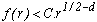In other words, f increases not as fast as the square root.
Then, for nearly all the number in the interval [0,1], by denoting ak the coefficients of their regular continue fraction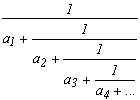, we have the following equality :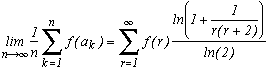The proof of this theorem, which takes several pages of the book by Khintchine, seems a bit too ambitious for this site, but if one day I have the courage, I will put it up maybe!
There exists other version based for example on ergodic theory, which is not that surprising seing the shape of the result.
We observe that the conditions on f is sufficient to make sure of the convergencce of the element on the right whose term in the serie at infinity is equivalent to f(r)/r2.
We now take a function f which satisfy the hypothesis of the thoerem, the logarithm.
We then get that for nearly all the numbers in [0,1] :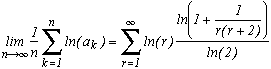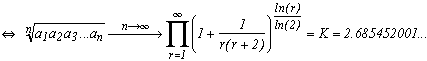And here appear the famous Khintchine's constant... It is a very interesting one by the way, but if we start going off topic, we'll never finish! Still see the reference of the bibliography to know a bit more.
Not easy to do calculation with this expression. But Bailey, Borwein and Crandall recently managed to get 7350 decimales of K with the help of the Zêta functions which converges a lot faster.
We can also obtain a formula with the harmonic mean and f(r)=r-1 :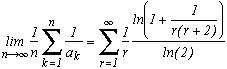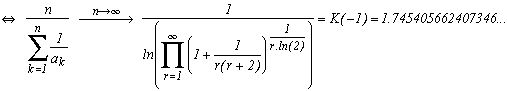We could also get a K(-2) by taking f(r)=r-2 etc....
This second formula for the harmonic mean is also a result "mostly true", so a small set of numbers ("negligible" for probability) donc satisfy this formula. Ok, all those fractions with coefficients very easily defined sequences will be part of this negligible set (think of the number e for example, or the golden ration, who trivially don't satisfy the two formulae of geometric or harmonic mean)... but what is the shape of the set of sequences such that the geometric mean don't tend toward the considered expression? It's a simple question of analysis, no?
And even if we know that numbers that don't follow this result are very rare, the idea is to check if the coefficient of the continu fraction of Pi do follow that result.
In all, another way to find a particular group to fit Pi into. Surronding it one way or the other!

David Bailey said to this that the coefficients of the continu fraction of Pi don't seems to follow a pattern and are supposed to be random in a certain way.

We now know 17 001 303 coefficients of the continu fraction of Pi of which the first are Pi = [3; 7; 15; 1; 292; 1; 1; 1; 2; 1; 3; ...]. The geometric mean of those coefficients is 2.686393 and the harmonic mean is 1.745882. The are approching the constant of K and K(-1) even if the calculation is done on only 17 millions coefficients, which is quite small compare to infinity !
So here again, no conclusion can be drawn...

2 - And so?

We can see with Khintchine's constant, taking the senic route through fascinating theories on the general behavious of numbers, mathematiciens tried to observed if Pi behaved in a particular way. The tools of statistic study of  decimals  being very limited and not having given much, it's a new very interesting approach.
The decimal quest will most probably not bear any fruits to this study parodoxly because there is no reason why after 207 billions of decimales, sundunly we find a pattern that is so obvious!! It's still the dream of many mathematicians after all, so you never know....
But the pattern is probably already in front of our eyes which are wide open, but either still not enough, or there doesn't exist one and we will never know if we can prove a really strong result such the normality of the decimals of Pi. And even, Pi would not be Pi without ITS own decimals, and not one different! The normality is not all, it doesn't trivialize completly and definitly the number.
Similarly for the representation of Pi in continu fraction. But this idea of representation is interesting. We have seen with the decount algorithm that there exists some bases where Pi is one of the simplest number, that is remarquable! The more ways we find to represent the numbers and the nnumber class associated with those representation, the more of an idea we will have of Pi and it's place with the numbers will be more precise. So for me it's still a very open domaine...

D - Bibliography

4 references of reference !

 Laboratoire de Kanada, Archives de Pi - Super-computing.org
ftp://pi.super-computing.org/

 Patrick Vanouplines, Université libre de Bruxelles, Rescaled range analysis and the fractal dimension of Pi.
http://gopher.ulb.ac.be/~pvouplin/pi/pi.ps

 D. Bailey, J. Borwein , R. Crandall, On the Khintchine Constant
http://www.nas.nasa.gov/Research/Reports/Techreports/1997/PDF/nas-97-030.pdf

 "La constante de Khintchine", miroir basé à l'Inria du site de Steven Finch
http://pauillac.inria.fr/algo/bsolve/constant/khntchn/khntchn.html